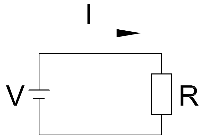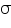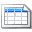# Back to Basics - Ohm’s Law

By on

## The Basics

Electrical engineering has a multitude of laws and theorems.  It is fair to say the Ohm's Law is one of the more widely known; if not the most known. Developed in 1827 by Georg Ohm the law defines the relationship between voltage, current and resistance in an electric circuit.

Voltage is the force that is used to drive current around an electric circuit.  This can be provided by batteries, generators, etc., is measured in Volts (V) and denoted by the symbol V.  The voltage drives electric current around the circuit and this current does the useful work (heating an element in a light bulb for example).  Electric current is  typically described as the flow of electrons around a circuit, is measured in Amperes (A) and denoted by the symbol I.   Resistance is the opposition an electric circuit provides to the flow of current;  it limits the amount of current flowing when a voltage is applied.  Resistance is measured in Ohms (Ω) and denoted by the symbol R.The illustration shows a simple circuit consisting of a supply voltage V and resistance R around which flows a current I.  Ohm's law provides the relationship between these quantities:

That’s it - really straight forward and simple.   In words we can say the relationship between voltage and current in a circuit is a constant - the resistance.

The law can of course to be rearranged to give each parameter:

## A little more complexity

The above is strictly true for direct current (dc) circuits.  In a dc circuit the current flows in one direction (positive to  negative) and is a constant value.  This changes in alternating current (ac) circuits - see the note article on Alternating Current Theory.  Because the current is constantly changing in an ac circuit, the magnetic fields are in motion and introduce additional constraints on the flow of current.  In addition to resistance we have a new parameter called reactance.  These combine into a quantity called impedance  which opposes the flow of electric current when a voltage is applied.

Like resistance the ac impedance is measured in Ohms (Ω) and is denoted by the symbol Z.   The good news is that impedance behaves like resistance and Ohm's Law still applies:

Note: when talking about dc circuits the quantities  (V, I and R) are scalar values.  In ac circuits these are vector quantities and the mathematics can be more complicated.

## Even more complexity

To fully round off the discussion on Ohm's Law, it should be noted that it can be reformulated to apply in a more general sense to many physics problems, other than it’s pure application to dc or ac circuits.  This reformulation was carried out by Gustav Kirchhoff and is:

In this representation J is the current density at some location (in a material or free space) and is measured in Ampere per metre squared.  E is the electric field in Volts per metre andis the conductivity of the material in Siemens per meter.  Conductivity is the inverse of resistivity (Ohm meter).

There are other representations of Ohm's Law, but these are starting to transgress into the realm of theoretical interest only.  If you are interested in an application of the above formulae, you can look at the Earth Electrode Resistance note.

Being such an important and fundamental electrical law, most of us have had the experience learning and applying Ohm’s law.  If you have any tips or other Ohm’s law goodies to share, please add these below.

More interesting Notes:Steven McFadyen

Steven has over twenty five years experience working on some of the largest construction projects. He has a deep technical understanding of electrical engineering and is keen to share this knowledge. About the author

Battery Sizing

This article gives an introduction to IEEE 485 method for the selection and calculation of battery capacity.

A mechanical engineering paper, some history and memories

I was digging in my bookshelf and came across the 80th Anniversary Association of Mine Resident Engineers, Papers and Discussions Commemorative Edition...

Why is electricity so hard to understand?

It's been a busy few months on different projects or busy couple of decades depending on how I look at it. I can say that on the odd (frequent) occasion...

Capacitor Theory

Capacitors are widely used in electrical engineering for functions such as energy storage, power factor correction, voltage compensation and many others...

Post Editing Tips

If you at all familiar with programs like office and outlook, then adding and editing posts is pretty straightforward and intuitive.  However, there are...

110 or 230 Volts

I've been considering a blog on the 110 or 230 Volt issue for a while.  While browsing the Internet I came across a great summary by Borat over at  engineering...

Restricted Earth Fault Protection

The windings of many medium and small sized transformers are protected by restricted earth fault (REF) systems. The illustration shows the principal of...

Motor Insulation

Insulation on a motor prevents interconnection of windings and the winding to earth.  When looking at motors, it is important to understand how the insulation...

E-Ink

Before the technical, some general information. E-ink display are found in a lot of e-readers, some mobile phones and similar devices and the intent is...

Cold Fusion (or not?)

Recently I have seen a few interesting articles on viable cold fusion; the combining of atoms at room like temperatures to create boundless energy. Now...## Have some knowledge to share

If you have some expert knowledge or experience, why not consider sharing this with our community.

By writing an electrical note, you will be educating our users and at the same time promoting your expertise within the engineering community.

To get started and understand our policy, you can read our How to Write an Electrical NoteClick here to view the notes list in a table formatClick here to view the notes indexed by tag